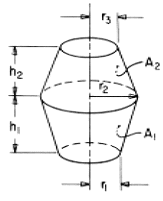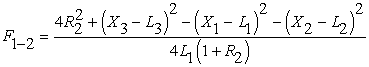# SECTION C Factors From Finite Areas to Finite Areas

## r2 > r3Definitions:
 H = h/r1; R = r/r1 L1 = [H12 + (R2 - 1)2]1/2; L2=[H22 + (R2 - R3)2]1/2 L3 = [(H1 + H2)2 + (R3 - 1)2]1/2 X1 = (L12 + 4R2)1/2 X2 = (L22 + 4R2R3)1/2 X3 = (L32 + 4R3)1/2

Governing Equation:To download a copy of this equation in MS Word 97 Format, click HERE.